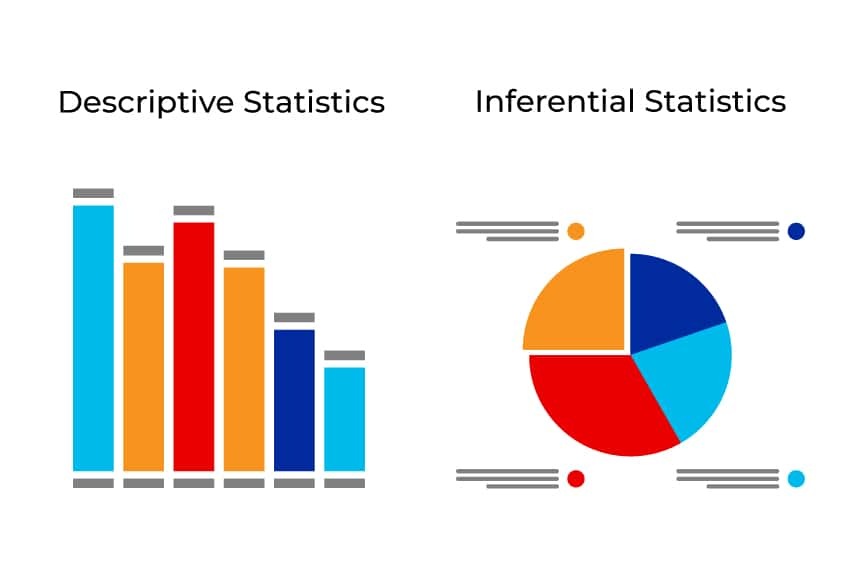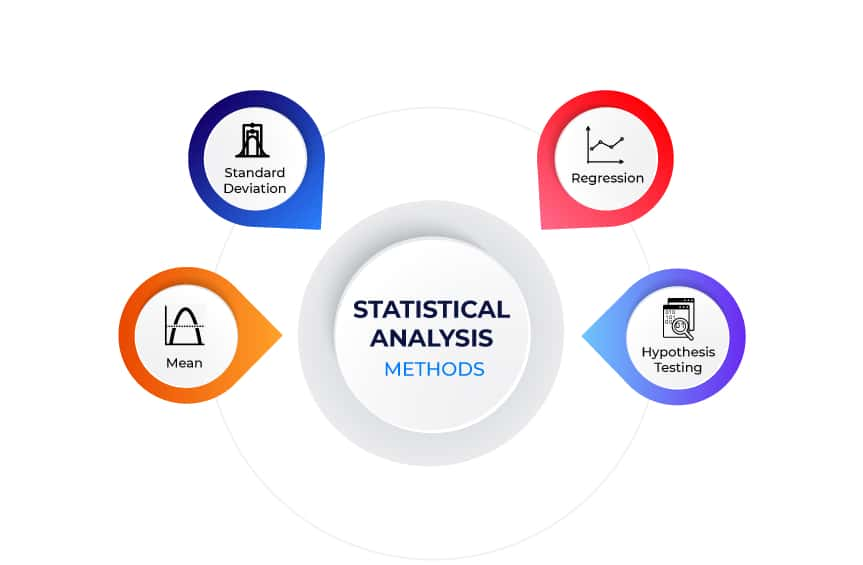# Statistical Analysis- Types, Methods & Examples

Statistics is an important part of Data Science where using Statistical Analysis, organisations can derive the value of the data input and evaluate meaningful conclusions. Accordingly, there are different types of statistical analysis techniques that are essential for application in various industries with a wide range of data. Moreover, it is important to know the different methods of statistical Analysis and the ways to use them for exploring data, finding patterns and identifying market trends. Read the following blog to find out more about What is Statistical Analysis and the different types and methods of statistical Analysis.

## What is Statistical Analysis?

Statistical Analysis refers to the procedure where business organisations make sense of large volumes of data and helps guide their decision-making process. Effectively, it is done on datasets where the Analysis creates different types of output based on the input data. For instance, statistical Analysis can summarise data, derive the values from the input data, provide input data characteristics, prove a null hypothesis, etc. The output varies based on the method of Analysis.

Statistical Analysis is crucial for governmental organisations as well as business management professionals. Moreover, it helps the experts within an organisation understand the complexities involved in a business scenario and the association of the data. Using statistical data, it is possible to generate information that derives political theory, campaign strategy and development of policies.

## Types of Statistical AnalysisSignificantly, there are two main types of Statistical Analysis- Descriptive Statistics and Inferential Statistics. The explanation of these two types is given below:

### Descriptive Statistics

The process of collecting, organising, analysing and summarising datasets and presenting them in an understandable form using graphs, charts and tables is known as Descriptive Statistics. Accordingly, with the help of Descriptive Statistics, it is possible to make large datasets presentable and eliminates major complexities for Data Analysts to analyse the data. The format of the summarised data can be quantitative or visual.

### Inferential Statistics

With the help of inferential statistics, it is possible to determine the inference of a large population group. Effectively, Inferential Statistics focuses on the Analysis and findings of the large sample data that is gained from a large population. Accordingly, it makes the process cost-efficient and time-saving as well. Furthermore, it includes the development of interval estimates and points estimates for conducting Analysis.

There are other types of Statistical Analysis as well which includes the following:

Predictive Analysis: Significantly, it is the type of Analysis useful for forecasting future events based on present and past data. Accordingly, it uses machine learning tools, data mining processes, big data, predictive modelling, artificial intelligence and simulations for Predictive Analysis.

Prescriptive Analysis: Significantly, the use of Prescriptive Analysis helps in prescribing the best possible outcome for assessing datasets. Moreover, it helps make informed decisions and encourages efficient decision-making processes.

Exploratory Data Analysis: Significantly, the use of exploratory data analysis in Statistics studies the datasets to highlight the major features of the data. Accordingly, the use of this type of Analysis is undertaken with statistical graphics or other visualization approaches.

Causal Analysis: Effectively, the use of casual Analysis is helpful in evaluating the cause and effect of a set of events. It implies that this type of Analysis focuses on analysing the issues of an event and identifies the reason behind them. Effectively, using Data Analytics, it is possible to analyse and find the reasons for unacceptable outcomes or failures in a business or business activities.

## Basic Methods of Statistical AnalysisSome of the Basic Methods of Statistical Analysis are as follows:

### Mean

Calculating the mean involves finding the sum of all the numbers in a list and dividing the answer by the total number of items in the list. Effectively, it is one of the simplest forms of Statistical Analysis, which allows a user to determine the central point in a dataset. Accordingly, the formula for calculating the mean is:

Mean = Set of numbers/ number of items.

### Standard Deviation

Standard Deviation is the method that helps determine how data can be spread around the mean, implying the dispersion of data points. Accordingly, a high standard deviation means that data dispersion is wider from the mean, and low standard Deviation implies that data is closer to the mean. To calculate standard Deviation, here is the formula you can use:

σ2 = Σ(x − μ)2/n

σ represents the Standard Deviation

Σ represents the sum of the data

x represents the value of the dataset

μ represents the mean of the data

n represents the number of data points in the population

### Regression

Regression is the method in Statistical Analysis which helps in finding the relationship between a dependent and independent variable. Significantly, it helps in tracking the changes in one variable and how it affects the changes in another. Accordingly, with the help of the regression method, it is possible to show whether the relationship between two variables is strong or weak or will vary over time. The regression formula is:

Y = a + b(x)

Where,

Y represents the independent variable

x represents the dependent variable

a represents the y-intercept or the value of y when x is equal to 0

b represents the slope of the regression graph.

### Hypothesis testing

Hypothesis Testing is the statistical analysis method where you test if a conclusion is valid for a specific dataset by comparing against a set of assumptions. Effectively, the test result can help nullify the hypothesis, in which case it becomes a null hypothesis or hypothesis 0. Additionally, anything that stands against or violates hypothesis 0 is the first hypothesis or hypothesis 1.

## Statistical Analysis Examples

### Statistical Analysis example using Mean Method

There are a total of 10 students in a class and the table below represents their results in the Maths test. Using the basic methods of Statistical Analysis. Find the mean of the data summarising the entire dataset to find the average score of the class.

 Students (S) Score out of 100 S1 40 S2 70 S3 75 S4 80 S5 85 S6 65 S7 78 S8 99 S9 97 S10 93

Mean = sum of a set of numbers/set of items

= 782/10

= 78.2

Hence, the mean score of the class in the Maths test for 10 students is 78.2.

### Statistical Analysis Example using Regression Method

Find the cost of maintaining a car driven for 50,000 miles if the maintenance cost when there is 0 mileage is \$100. Take b as 0.03, so the cost of maintenance increases by \$0.03 for every unit increase in miles driven.

Let,

Y = cost of car maintenance

x = 50,000 miles

a= \$100

b = \$0.03

Using the Regression formula,

Y = a + b(x)

Y = \$100 + 0.03(50,000)

Y= \$1600

The above value shows that the mileage is able to affect the maintenance cost of a car.

## Conclusion

Hence, Statistical Analysis is a crucial part of Data Science and the different types and methods of Statistical Analysis. Moreover, Data Scientists use different methods and techniques to analyse datasets within organisations where statistical inferences become important. Effectively, while it is not necessary for you to have a statistical background to become a Data Scientist or Data Analyst, developing statistical skills will only make you more efficient in the field.

### Asmita Kar

I am a Senior Content Writer working with Pickl.AI. I am a passionate writer, an ardent learner and a dedicated individual. With around 3years of experience in writing, I have developed the knack of using words with a creative flow. Writing motivates me to conduct research and inspires me to intertwine words that are able to lure my audience in reading my work. My biggest motivation in life is my mother who constantly pushes me to do better in life. Apart from writing, Indian Mythology is my area of passion about which I am constantly on the path of learning more.